# linear model

### Member Training: The Link Between ANOVA and Regression

January 31st, 2023 byIf you’ve used much analysis of variance (ANOVA), you’ve probably heard that ANOVA is a special case of linear regression. Unless you’ve seen why, though, that may not make a lot of sense. After all, ANOVA compares means between categories, while regression predicts outcomes with numeric variables.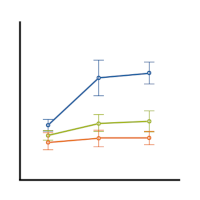(more…)

### The Difference Between Link Functions and Data Transformations

September 24th, 2018 by

Generalized linear models—and generalized linear mixed models—are called generalized linear because they connect a model’s outcome to its predictors in a linear way. The function used to make this connection is called a link function. Link functions sounds like an exotic term, but they’re actually much simpler than they sound.

For example, Poisson regression (commonly used for outcomes that are counts) makes use of a natural log link function as follows: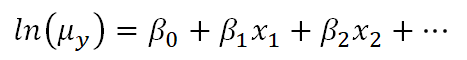Clearly, there is not a direct linear relationship of the x variables to the average count, but there is a “sort of linear” relationship happening: a function of the mean of y is related to a linear combination of x variables. In other words, the linear model has now been generalized to a bigger type of situation.

This can lead to confusion, though, because on the surface it looks very similar to what happens when we transform the dependent variable in a linear model, like a linear regression.

The key thing to understand is that the natural log link function is a function of the mean of y, not the y values themselves.

### Transformations of Y

Below is a linear model equation where the original dependent variable, y, has been natural log transformed. That is, the natural log has been taken of each individual value of y, and that is being used as the dependent variable.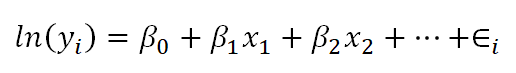The linear model with the log transformation is providing an equation for an individual value of ln(y). We could also write it as follows, where we are modeling the mean of ln(y) (note the error term is no longer present):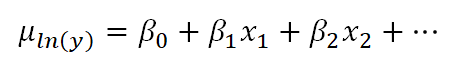This makes the difference a bit clearer. When we transform the data in a linear model, we are no longer claiming that y is normally distributed around a mean, given the x values — we are claiming that our new outcome variable, ln(yi), is normally distributed.

In fact, we often make this transformation specifically because the values of y do not appear to be normally distributed around their average.

In the case of the Poisson model, however, the link function does not change the distribution of the actual observations in some way to make them something other than Poisson distributed. Instead, the link function defines the relationship of the x variables directly to the mean of the Poisson distributed y. The individual observations then vary around this expected value accordingly.

### The mean of the log is not the log of the mean

As you may know, if you have used this kind of data transformation in a linear model before, you cannot simply take the exponent of the mean of ln(y) to get the mean of y.

You might be surprised to know, though, that you can do this with a link function. If you have specific values of your x variables, you can calculate the predicted average count, μy based on those x values by inversing the natural log:This ability to back-transform means (and regression coefficients) to a more intuitive scale is part of what makes generalized linear models so useful.

### Member Training: Generalized Linear Models

September 3rd, 2018 by
In this webinar, we will provide an overview of generalized linear models. You may already be using them (perhaps without knowing it!).For example, logistic regression is a type of generalized linear model that many people are already familiar with. Alternatively, maybe you’re not using them yet and you are just beginning to understand when they might be useful to you.

### Using Pairwise Comparisons to Help you Interpret Interactions in Linear Regression

January 12th, 2018 by

In a previous post we discussed using marginal means to explain an interaction to a non-statistical audience. The output from a linear regression model can be a bit confusing. This is the model that was shown.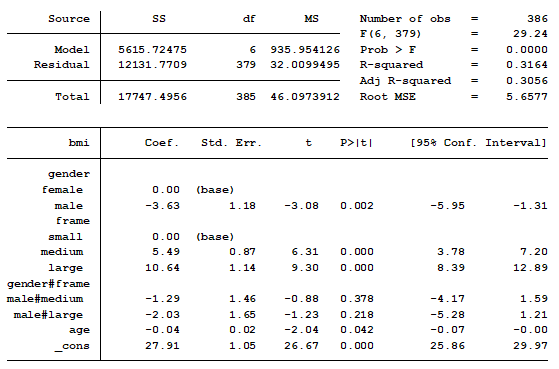In this model, BMI is the outcome variable and there are three predictors:

### What Are Nested Models?

July 28th, 2017 by

Pretty much all of the common statistical models we use, with the exception of OLS Linear Models, use Maximum Likelihood estimation.

This includes favorites like:

That’s a lot of models.

If you’ve ever learned any of these, you’ve heard that some of the statistics that compare model fit in competing models require (more…)

### Incorporating Graphs in Regression Diagnostics with Stata

May 24th, 2016 byYou put a lot of work into preparing and cleaning your data. Running the model is the moment of excitement.

You look at your tables and interpret the results. But first you remember that one or more variables had a few outliers. Did these outliers impact your results? (more…)